# Clock

How long is trajectory of second hand of hours for day, if is 15 mm long?

l =  135.7 m

### Step-by-step explanation: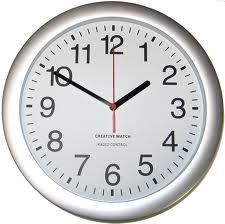We will be pleased if You send us any improvements to this math problem. Thank you!Tips to related online calculators
Do you want to convert length units?

#### You need to know the following knowledge to solve this word math problem:

We encourage you to watch this tutorial video on this math problem:

## Related math problems and questions:

• The small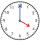The small hand of the wall clock is 15 cm long. What a long journey the end of the hour hand in 15 minutes will describe. (b - in 2 hours)?
• Clock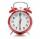What distance will pass end of 8 cm long hour hand for 15 minutes?
• Minute handWhat is the distance the minute hand of the clock travels in 12 minutes, if the diameter of the clock is 30 cm and the hand extends to a distance of 2 cm from the edge of the clock?
• Clock handsThe second hand has a length of 1.5 cm. How long does the endpoint of this hand travel in one day?
• ClocksHow long track travel the second hand of a clock in 46 hours, which is 4 cm long.
• Minute-hand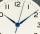How long distance will travel a large (minute) hand on the clock for 48 minutes, if its length is 56 cm?
• ClocksHow will long path walk the end of the clock's minute-hand long 7 cm for 330 minutes? How long will walk in the same time hour-hand?
• LunchLunch is given to seniors from 12:15 to 12:40 during the Coronavirus pandemic. What angle will the minute hand of clock describe during this time?
• ClocksThe length of the minute hand is 12 cm. What track in meters will its end point describe in a week?
• HandsThe clock shows 12 hours. After how many minutes will angle between the hour and minute hand 90°? Consider the continuous movement of both hands hours.
• Rectangle and circle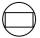The rectangle ABCD has side lengths a = 40 mm and b = 30 mm and is circumscribed by a circle k. Calculate approximately how many cm is circle long.
• The big clock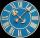The big clock hands stopped at a random moment. What is the probability that: a) a small hand showed the time between 1:00 and 3:00? b) the big hand was in the same area as a small hand in the role of a)? c) did the hours just show the time between 21:00
• Clock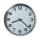How many hours are 15 days?
• Clock Tower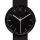What angle is betwenn hands on Clock Tower when show 17 hours and 35 minutes?
• The central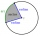The central angle of a sector is 30° and the radius is 15 m. Determine its perimeter.
• ClockHow many times a day hands on a clock overlap?
• Time clockWhat are the angle of the hour hand and the minute hand if it is 0:40?